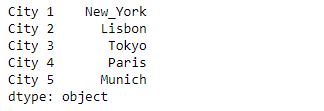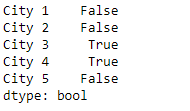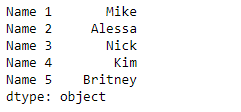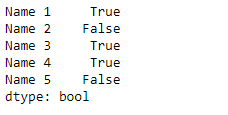# Python | Pandas Series.str.match()

• Last Updated : 27 Mar, 2019

`Series.str` can be used to access the values of the series as strings and apply several methods to it. Pandas` Series.str.match()` function is used to determine if each string in the underlying data of the given series object matches a regular expression.

Syntax: Series.str.match(pat, case=True, flags=0, na=nan)

Parameter :
pat : Regular expression pattern with capturing groups.
case : If True, case sensitive
flags : A re module flag, for example re.IGNORECASE.
na : default NaN, fill value for missing values

Returns : Series/array of boolean values

Example #1: Use `Series.str.match()` function to match the passed regular expressions with the string in the underlying data of the given series object.

 `# importing pandas as pd``import` `pandas as pd`` ` `# importing re for regular expressions``import` `re`` ` `# Creating the Series``sr ``=` `pd.Series([``'New_York'``, ``'Lisbon'``, ``'Tokyo'``, ``'Paris'``, ``'Munich'``])`` ` `# Creating the index``idx ``=` `[``'City 1'``, ``'City 2'``, ``'City 3'``, ``'City 4'``, ``'City 5'``]`` ` `# set the index``sr.index ``=` `idx`` ` `# Print the series``print``(sr)`

Output :Now we will use `Series.str.match()` function to match the passed regular expressions with the string in the underlying data of the given series object.

 `# match either 'Tokyo' or 'Paris'``result ``=` `sr.``str``.match(pat ``=` `'(Tokyo)|(Paris)'``)`` ` `# print the result``print``(result)`

Output :As we can see in the output, the `Series.str.match()` function has returned a series of boolean values. It contains `True` for those values which successfully matches else it contains `False`.

Example #2 : Use `Series.str.match()` function to match the passed regular expressions with the string in the underlying data of the given series object.

 `# importing pandas as pd``import` `pandas as pd`` ` `# importing re for regular expressions``import` `re`` ` `# Creating the Series``sr ``=` `pd.Series([``'Mike'``, ``'Alessa'``, ``'Nick'``, ``'Kim'``, ``'Britney'``])`` ` `# Creating the index``idx ``=` `[``'Name 1'``, ``'Name 2'``, ``'Name 3'``, ``'Name 4'``, ``'Name 5'``]`` ` `# set the index``sr.index ``=` `idx`` ` `# Print the series``print``(sr)`

Output :Now we will use `Series.str.match()` function to match the passed regular expressions with the string in the underlying data of the given series object.

 `# match groups having any capital letter``# followed by 'i' and any other character``result ``=` `sr.``str``.match(pat ``=` `'([A-Z]i.)'``)`` ` `# print the result``print``(result)`

Output :As we can see in the output, the `Series.str.match()` function has returned a series of boolean values. It contains `True` for those values which successfully matches else it contains `False`.

My Personal Notes arrow_drop_up